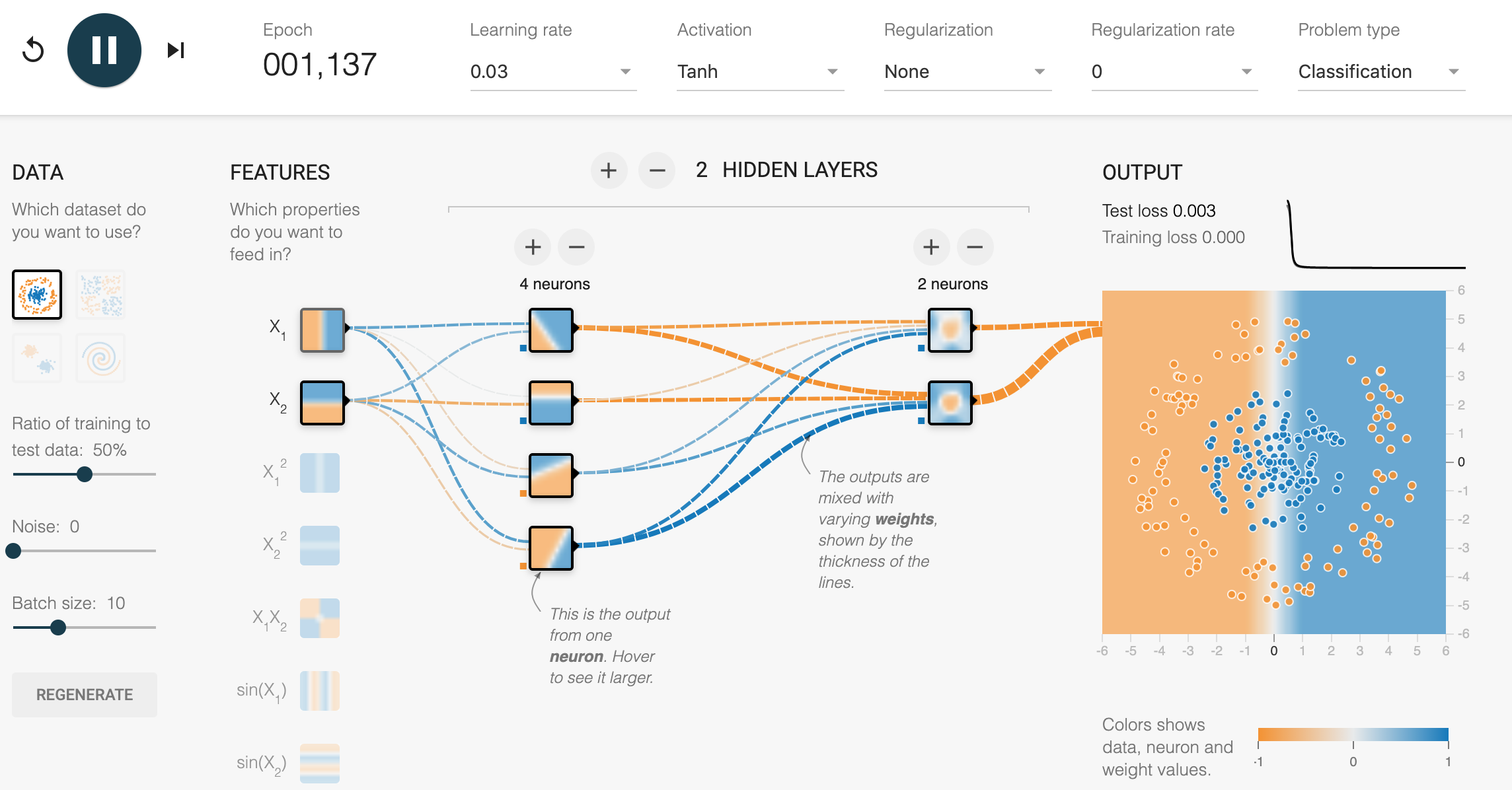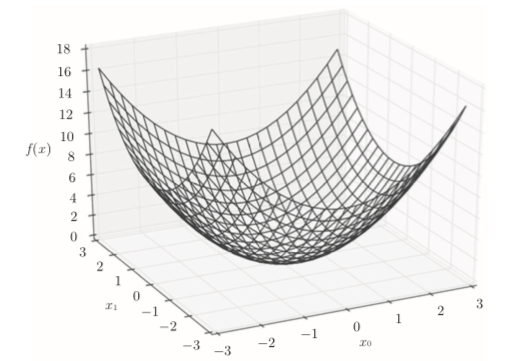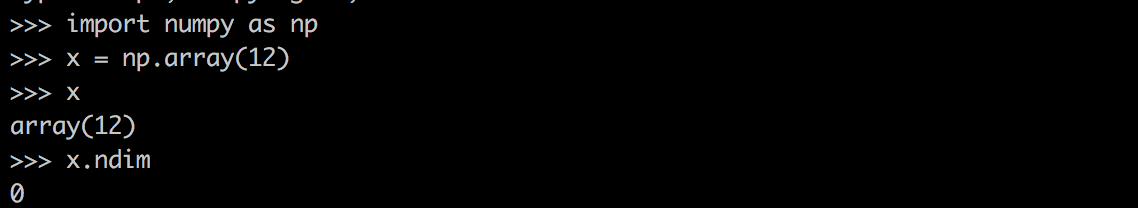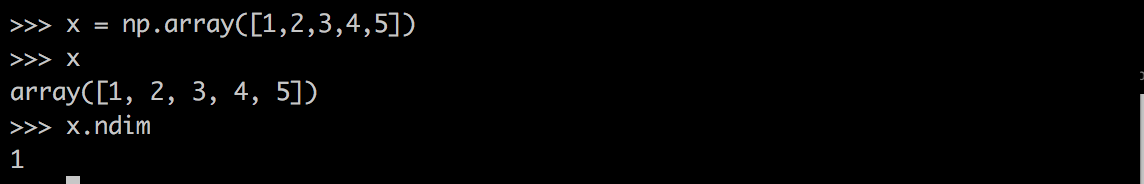神经张量网络_张量神经网络 - CSDN

• 欢迎大家前往云加社区，获取更多腾讯海量技术实践干货哦~ 译者：Waitingalone 本文翻译自Gaurav Bhatt在 http://deeplearn-ai.com ...文中版权、图像代码等数据均归作者所有。为了本土化，翻译内容略作修改。 ......


欢迎大家前往云加社区，获取更多腾讯海量技术实践干货哦~

译者：Waitingalone
本文翻译自Gaurav Bhatt在 http://deeplearn-ai.com 发表的NEURAL TENSOR NETWORK: EXPLORING RELATIONS AMONG TEXT ENTITIES。文中版权、图像代码等数据均归作者所有。为了本土化，翻译内容略作修改。

在这篇文章中，我将介绍神经张量网络（NTN），如在用神经张量网络推理知识库的推理中所描述的那样 。我的NTN实现使用最新版本的Python 2.7，Keras 2.0和Theano 0.9。
用代码直接跳到GitHub仓库。
什么是知识库完成？
在知识库完成中，任务是确定两个实体对之间的关系。例如，考虑两个实体对 -<cat, tail> 和<supervised learning, machine learning>。如果我们被要求确定给定的两对之间的关系 - <cat，R，tail>和<supervised learning, R, machine learning> - 那么第一个关系可以最好的归结为有型，而第二个关系可以被归结为实例。所以，我们可以将这两个对重新定义为 <cat，has，tail>和<supervised learning，instance of，machine learning>。神经张量网络（NTN）在实体 - 关系对的数据库上训练，用于探究实体之间的附加关系。这是通过将数据库中的每个实体（即每个对象或个体）表示为一个向量来实现的。这些载体可以捕获有关该实体的事实，以及它是如何可能是某种关系的一部分。每个关系都是通过一个新的神经张量网络的参数来定义的，这个神经张量网络可以明确地涉及两个实体向量使用NTN预测新的关系三元组。
关系推理的神经模型
能够认识到某些事实纯粹是由于其他现有的关系而存在的，是学习常识推理的模型的目标。NTN旨在发现实体<e1,e2>之间的关系，即对于<e1，e2>确定性地预测关系R. 例如，(e1,R,e2) = (Bengal tiger, has part, tail) 这个关系是否真实且具有确定性。神经张量网络（NTN）用一个双线性张量层代替一个标准的线性神经网络层，它直接关联了多个维度上的两个实体向量。该模型通过下列基于NTN的函数计算两个实体处于特定关系的可能性分数：其中是标准非线性的单元应用,是张量，双线性张量积产生向量，其中每个条目张量的一个切片计算：。其它参数为关系R是一个神经网络的标准形式：和，可视化神经张量层NTN使用张量变量对两个实体之间的关系进行乘法建模。如上所示，NTN是对简单神经层的扩展，增加了这些张量变量。所以，如果我们从上图中删除，最后，目标函数被定义为这是一个简单的实体向量连接，以及偏向项。
培训目标
NTN采用对比式最大余量目标函数进行训练。给定训练样本中的三元组，则通过随机的将第二个实体替换为来创建负样本，其中j是随机索引。最后，目标函数被定义为其中，是正则化参数。
实施细节
现在，我们看到了NTN的工作，是时候深入实施了。这里要考虑的重要一点是，每个给定的关系都有其自己的一组张量参数。让我简单介绍一下在Keras的帮助下我们需要做些什么。每个关系都归因于一个单独的Keras模型，它也增加了张量参数。现在，假定张量层是在模型初始化和组合之间添加的。在后面的文章中，我将解释张量层的构造。从上图可以很容易得出结论，我们需要以某种方式处理训练数据，以便它可以同时传递到所有单独的模型。我们想要的只是更新与特定关系相对应的张量参数。然而，Keras 并没有让我们更新一个单独的模型，而剩下的。所以我们需要把数据分成不同的关系。每个训练样本将包含所有关系的一个实例，也就是每个关系的一对实体。
实施NTN层
让我们从实施神经张量层开始。这部分的先决条件是在Keras编写自定义图层。如果您不确定这意味着什么，那么请查看Keras文档的 编写你自己的keras图层。
我们首先用参数inp_size，out_size和activation来初始化NTN类。该inp_size是输入变量的形状，在我们的例子中的实体; 所述out_size是张量参数（K），和激活是要使用的激活函数（默认为tanh）。

from ntn_input import *
from keras import activations

class ntn_layer(Layer):
def __init__(self, inp_size, out_size, activation='tanh', **kwargs):
super(ntn_layer, self).__init__(**kwargs)
self.k = out_size
self.d = inp_size
self.activation = activations.get(activation)
self.test_out = 0

维的命名保持不变，即k对应于每个关系的张量参数个数，d是实体的形状。
现在，我们需要初始化张量图层参数。为了更好的理解我们在这里做什么，看一下张量网络的下图。我们初始化四个张量参数，即W，V，b和U如下：

def build(self,input_shape):
self.W = self.add_weight(name='w',shape=(self.d, self.d, self.k), initializer='glorot_uniform', trainable=True)

self.V = self.add_weight(name='v', shape=(self.k, self.d*2), initializer='glorot_uniform', trainable=True)

self.b = self.add_weight(name='b', shape=(self.k,), initializer='zeros', trainable=True)

super(ntn_layer, self).build(input_shape)

一旦参数被初始化，那么是时候实现下面的等式了：上面的等式给出了每个实体对的分数。正如你所看到的，我们必须迭代k个张量参数（张量模型的切片）。这是通过计算每个迭代的中间产品来完成的，最后，汇总所有这些产品。下面的代码片段为你做这个。请不要更改函数的名称，因为它们与Keras API一致。

e1=x # 实体 1
e2=x # 实体 2
batch_size = K.shape(e1)
V_out, h, mid_pro = [],[],[]
for i in range(self.k): # 计算内部产品
V_out = K.dot(self.V[i],K.concatenate([e1,e2]).T)
temp = K.dot(e1,self.W[:,:,i])
h = K.sum(temp*e2,axis=1)
mid_pro.append(V_out+h+self.b[i])

tensor_bi_product = K.concatenate(mid_pro,axis=0)
tensor_bi_product = self.U*self.activation(K.reshape(tensor_bi_product,(self.k,batch_size))).T

self.test_out = K.shape(tensor_bi_product)
return tensor_bi_product

最后，要完成NTN层的实现，我们必须添加以下功能。这与NTN无关; Keras使用以下函数进行内部处理。

def compute_output_shape(self, input_shape):
return (input_shape,self.k)

我们已经建立了可以像Keras中的任何其他神经层一样调用的NTN层。让我们看看如何在真实的数据集上使用NTN层。
数据集
我将使用文中提到的Wordbase和Freebase数据集。我已经准备好了数据集（预处理的一部分从GitHub存储库中获取），并且可以进行如下处理。

import ntn_input

data_name = 'wordbase' # 'wordbase' or 'freebase'
data_path = 'data'+data_name
indexed_training_data = data_to_indexed(raw_training_data, entities_list, relations_list)
indexed_dev_data = data_to_indexed(raw_dev_data, entities_list, relations_list)

num_entities = len(entities_list)
num_relations = len(relations_list)

此时您可以打印并查看实体及其对应的关系。现在，我们需要根据关系来划分数据集，以便所有Keras模型都可以同时更新。我已经包括一个预处理功能，为您执行此步骤。此步骤中还添加了否定样本。负样本作为损坏的样本传递给prepare_data函数。如果corrupt_samples = 1，则对应于每个训练样本添加一个负样本。这意味着，整个训练数据集将会翻倍。
import ntn_input
e1,e2,labels_train,t1,t2,labels_dev,num_relations = prepare_data(corrupt_samples)
NTN的定义存储在一个名为ntn的文件中，很容易导入使用。
建立模型
为了训练模型，我们需要定义对比最大边缘损失函数。

def contrastive_loss(y_true, y_pred):
margin = 1
return K.mean(y_true * K.square(y_pred) + (1 - y_true) * K.square(K.maximum(margin - y_pred, 0)))

我们应该可以从Keras编译函数中调用这个自定义的丢失函数。

from ntn import *

def build_model(num_relations):
Input_x, Input_y = [], []
for i in range(num_relations):
Input_x.append(Input(shape=(dimx,)))
Input_y.append(Input(shape=(dimy,)))

ntn, score = [], [] # 存储单独的张量参数
for i in range(num_relations): # 通过每个切片迭代 'k'
ntn.append(ntn_layer(inp_size=dimx, out_size=4)([Input_x[i],Input_y[i]]))
score.append(Dense(1,activation='sigmoid')(ntn[i]))

all_inputs = [Input_x[i]for i in range(num_relations)]
all_inputs.extend([Input_y[i]for i in range(num_relations)]) # 聚合所有模型

model = Model(all_inputs,score)
return model

最后，我们需要汇总数据以训练模型

e, t, labels_train, labels_dev = aggregate(e1, e2, labels_train, t1, t2, labels_dev, num_relations)
model.fit(e, labels_train, nb_epoch=10, batch_size=100, verbose=2)

在这一点上，你可以看到模型开始训练，每个个体模型的损失逐渐减少。此外，为了计算NTN在知识库数据集上的准确性，我们需要计算所有关系的成本，并选择最大分数的成本。正如本文所述，所达到的准确度接近88％（平均）。
下一步是什么？
在这篇文章中，我们看到了建立知识库完成的神经张量网络。在下一篇文章中，我们将看到NTN如何用于解决其他NLP问题，例如基于非事实问题的回答。。

原文链接：http://deeplearn-ai.com/2017/11/21/neural-tensor-network-exploring-relations-among-text-entities/
原文作者：Gaurav Bhatt

相关阅读

推荐算法之协同过滤

实习生的监控算法: 利用时间序列模型进行曲线预测

用R和Keras深度学习的例子

此文已由作者授权云加社区发布，转载请注明原文出处

转载于:https://www.cnblogs.com/qcloud1001/p/8074771.html
展开全文阅读上一篇 深度学习的“Hello World”
开篇张量
神经网络使用的数据存储在多维Numpy 数组中，也叫张量（tensor），所以张量其实就是多维数组，所以不能叫做矩阵，矩阵只是二维的数组，张量所指的维度是没有限制的。一般来说，当前所有机器学习系统都使用张量作为基本数据结构。那么什么是张量？张量这一概念的核心在于，它是一个数据容器。它包含的数据几乎总是数值数据，因此它是数字的容器。你可能对矩阵很熟悉，它是二维张量。张量是矩阵向任意维度的扩展。下面对0到3维张做一些描述和实验，以加深理解。
注意，张量的维度（dimension）通常叫作轴（axis）
1、标量(scalar)
仅包含一个数字的张量叫作标量（scalar，也叫标量张量、零维张量、0D 张量）。在Numpy中，一个float32 或float64 的数字就是一个标量张量（或标量数组）。你可以用ndim 属性来查看一个Numpy 张量的轴的个数。标量张量有0 个轴（ndim=0）。张量轴的个数也叫作阶（rank）。
2、向量(vector)
数字组成的数组叫作向量（vector）或一维张量（1D张量）。一维张量只有一个轴。
3、矩阵(matrix)
向量组成的数组叫作矩阵（matrix）或二维张量（2D 张量）。矩阵有2个轴（通常叫作行和列）。你可以将矩阵直观地理解为数字组成的矩形网格。
4、3D张量与更高维张量
将多个矩阵组合成一个新的数组，可以得到一个3D 张量，你可以将其直观地理解为数字组成的立方体。将多个3D 张量组合成一个数组，可以创建一个4D 张量，以此类推。深度学习处理的一般是0D 到4D 的张量，但处理视频数据时可能会遇到5D 张量。
张量的关键属性
张量是由以下三个关键属性来定义的:

轴的个数（阶）。例如，3D 张量有3个轴，矩阵有2个轴。这在 Numpy 等 Python 库中也叫张量的ndim。
形状(shape)。这是一个整数元组，表示张量沿每个轴的维度大小（元素个数）。例如，前面矩阵示例的形状为(3, 5)，3D 张量示例的形状为(3, 3, 5)。向量的形状只包含一个元素，比如(5,)，而标量的形状为空，即()。
数据类型（在 Python 库中通常叫作 dtype）。这是张量中所包含数据的类型，例如，张量的类型可以是float32、uint8、float64 等。在极少数情况下，你可能会遇到字符（char）张量。注意，Numpy（以及大多数其他库）中不存在字符串张量，因为张量存储在预先分配的连续内存段中，而字符串的长度是可变的，无法用这种方式存储。

练习
把一个数不断的加[]让它变成多维数组，以及演示如何取数组的元素。
>>> data = np.array(12)
>>> print(data.ndim, data.shape,data)
0 () 12
>>> data = np.array([data])
>>> print(data.ndim, data.shape,data)
1 (1,) 
>>> data = np.array([data,])
>>> print(data.ndim, data.shape,data)
2 (2, 1) []
>>> data = np.array([data,data,data])
>>> print(data.ndim, data.shape,data)
3 (3, 2, 1) [[][][]]
>>> data.ndim, data.shape,data
(3, (3, 2, 1), array([[,],[,],[,]]))
>>> data
array([,])
>>> data
array([,])
>>> data
array([,])
>>> data
Traceback (most recent call last):
File "<stdin>", line 1, in <module>
IndexError: index 1 is out of bounds for axis 0 with size 1
>>> data
13

梯度
为了很好的理解梯度，我们先来回顾一下导数，偏导数的定义
导数
在高等数学中学过导数，导数就是表示某个瞬间的变化量，它可以定义成下面的式子：
$\frac{df(x)}{dx}=\lim_{x \to 0}\frac{f(x+h)-f(x)}{h}$
利用微小的差分求导数的过程称为数值微分(numerical  differentiation)。
偏导数
有多个变量的函数的导数称为偏导数，比如一个有两个变量的函数：
$f(x0,x1) = x0^2 + x1^2$
在某一个具体的点（3，4），就有两个导数，用数学表达式表示如下：$\frac {\theta f}{\theta x0},\frac {\theta f}{\theta x1}$
该函数图像如下：梯度
我们按变量分别计算了x0 和 x1的偏导数。现在，我们希望一起计算x0和x1的偏导数。比如，我们来考虑求x0 =3,x1 =4时的偏导数：$(\frac {\theta f}{\theta x0},\frac {\theta f}{\theta x1})$
这样由所有变量的偏导数汇总而成的向量成为梯度。可以看出梯度实质是一个张量，内容是所有变量在某点的偏导数的集合，只不过使用张量一次性方便描述和计算。
练习
为量巩固对导数、偏导数、梯度的理解和认识，练习时最好的方式，以下是练习的全部代码，根据需要打开 Test Case部分的注释，代码已经自带解释了。
# coding: utf-8
import matplotlib.pylab as plt
import numpy as np

def numerical_diff(self, f, x):
"""
函数求导数,实例是求1个自变量的函数
:param f: 传递的参数可以是一个函数
:param x: x 可以是多维数组，代表多个点
:return: 返回x中点的导数，值是跟x.shape相同的数组
"""
h = 1e-4  # 0.0001
return (f(x + h) - f(x - h)) / (2 * h)

"""
求导数
:param f:
:param X: 可以是多维数组
:return: 返回X.shape的导数值
"""
if X.ndim == 1:
else:

# 维度上循环
for idx, x in enumerate(X):
# print('{}->{}'.format(idx, x))

"""
返回一行的导数值
:param f: 一个自变量函数
:param x: x 可以是一维数组
:return:
"""
h = 1e-4  # 0.0001

for idx in range(x.size):
tmp_val = x[idx]
x[idx] = float(tmp_val) + h
# 这是把整个x变量带入函数，x里只有x[idx]值变了
fxh1 = f(x)  # f(x+h)

x[idx] = tmp_val - h
fxh2 = f(x)  # f(x-h)
grad[idx] = (fxh1 - fxh2) / (2 * h)

x[idx] = tmp_val

def tangent_line(self, f, x):
"""
求切线函数
:param f: 原来函数
:param x: 选的x点
:return: 一个函数(形式 kx+b)
"""
d = self.numerical_diff(f, x)
y = f(x) - d * x

return lambda t: d * t + y

def function_1(x):
return 0.01 * x ** 2 + 0.1 * x

def function_2(x):
if x.ndim == 1:
return np.sum(x ** 2)
else:
return np.sum(x ** 2, axis=1)

if __name__ == '__main__':

# # Test Case 1: 自变量只有一个的情况
# # 传入参数说明，传入1个值，返回一个值的导数，传入数组就返回数值中
# # 每个值处的导数，这是Numpy看起来有点神奇的地方。
# print(d2g.numerical_diff(function_1, 2.5))
# print(d2g.numerical_diff(function_1, np.array([2.5])))
# print(d2g.numerical_diff(function_1, np.array([2.5, 4, 5])))
# print(d2g.numerical_diff(function_1, np.array([[2.5, 3, 5, 6], [12, 2.5, 4, 3]])))
#
# x = np.arange(0.0, 20.0, 0.1)
# y = function_1(x)
# plt.xlabel("x")
# plt.ylabel("f(x)")
#
# tf = d2g.tangent_line(function_1, 5)
# y2 = tf(x)
#
# # 绘制f(x)函数和切线，这里要理解函数可以作为
# # 参数传递的技巧，再一次展现Python作为动态语
# # 言的一个优势。
# plt.plot(x, y)
# plt.plot(x, y2)
# plt.show()

# Test Case 2:
x0 = np.arange(-2, 2.5, 1)
x1 = np.arange(-1, 3.5, 1)
# 返回两个数组组成的数组，一个数组[x1.size * x0.size].
# 每行是x0所有数值；第二个数组是[x1.size * x0.size],
# 每1行的值是x1第2行值是x1,以此类推。
X, Y = np.meshgrid(x0, x1)
X = X.flatten()
Y = Y.flatten()
points = np.array([X, Y])

c = np.random.randn(len(X))  # arrow颜色

plt.figure()
plt.xlim([-2, 2])
plt.ylim([-2, 2])
plt.xlabel('x0')
plt.ylabel('x1')
plt.grid()
plt.legend()
plt.draw()
plt.show()

继续下一篇阅读 神经网络的数学基础：张量运算


展开全文机器学习 深度学习 梯度 神经网络
• 神经网络使用的数据存储在多维Numpy数组中，也叫张量（tensor）。 张量是一个数据容器，张量的维度（dimension）通常叫做轴（axis）。 1. 标量（0D张量） 仅含一个数字的张量叫做标量（scalar，也叫标量张量、零维...


神经网络使用的数据存储在多维Numpy数组中，也叫张量（tensor）。
张量是一个数据容器，张量的维度（dimension）通常叫做轴（axis）。
1. 标量（0D张量）
仅含一个数字的张量叫做标量（scalar，也叫标量张量、零维张量、0D张量）。在Numpy中，一个float32或float64数字就是一个标量张量（或标量数组）。可以用ndim属性来查看一个Numpy张量的轴的个数。标量张量有0个轴（ndim == 0）。张量轴的个数也叫做阶（rank）。
eg：2. 向量（1D张量）
数字组成的数字叫做向量（vector）或一维张量（1D张量）。
eg：这个向量有5个元素，所以被称为5D向量。
注：5D向量和5D张量不同，5D向量只有一个轴，沿着轴有5个维度，而5D张量有5个轴，沿着每个轴可能有任意个维度
维度（dimensionality）可以表示沿着某个轴上的元素个数，也可以表示张量中轴的个数。
3. 矩阵（2D张量）
向量组成的数组叫矩阵（matrix）或二维张量（2D张量）。矩阵有2个轴（也叫行和列）。
4. 3D张量与更高维张量
将多个矩阵组成一个新的数组，可以得到一个3D张量。
将多个3D张量合成一个数组，可以创建一个4D张量。
以此类推，深度学习处理的一般是0D到4D的张量，但处理视频数据时可能会遇到5D张量。
5. 关键属性
1）轴的个数（阶）
例如，3D张量有3个轴，矩阵有2个轴，这在Numpy等python库中也叫做张量的ndim。
2）形状
整数元组，表示张量沿每个轴的维度大小（元素个数）。例如，矩阵的形状可以为(3,5)，3D张量的形状可以为(3,3,5)。向量的形状只包含一个元素，比如(5,)，而标量的形状为空，即()。
3）数据类型（在python库中通常叫做dtype）
例如，张量的类型可以是float32、unit8、float64等。
6. 现实世界中的数据张量
1）向量数据
2D张量，形状为 (samples, features)。
2）时间序列数据或序列数据
3D张量，形状为 (samples, timesteps, features)。
3）图像
4D张量，形状为 (samples, height, width, channels) 或 (samples, channels, height, width)。
4）视频
5D张量，形状为 (samples, frames, height, width, channels) 或 (samples, frames, channels, height, width)。
7. 广播
1）向较小的张量添加轴（广播轴），使其ndim与较大的张量相同。
2）将较小的张量沿着新轴重复，使其形状与较大的张量相同。


展开全文机器学习 python 神经网络
• 在本文中，我们提出了一种新颖的深度关注神经张量网络（DA-NTN）用于视觉问题回答，它可以发现基于张量表示的图像，问题和答案之间的联合相关性。 首先，我们通过双线性特征对成对交互（例如，图像和问题）中的一个...
• 神经网络使用的数据存储在多维Numpy数组中，也叫张量（tensor）。 张量是一个数据容器，张量的维度（dimension）通常叫做轴（axis）。 1. 标量（0D张量） 仅含一个数字的张量叫做标量（scalar，也叫标量张量、零维...深度学习 神经网络
• 张量分解系列： 张量分解（一）：张量分解（一）：基础知识 ...Tensorizing Neural Network即张量化神经网络，跟一般神经网络的区别是把输入输出，权重，偏置等转换成张量的形式，反向传播同样使用链式法则。 这...张量分解
• 代码前戏大概前两章就是写了传统的神经网络对计算的要求有多高balabala，以及一些张量张量分解的发展等等。下面的张量神经网络会用到tt分解。tt分解的作者也是这篇文章的作者。part3第三章开始正片。 这部分就...神经网络 压缩
• ## TensorFlow中tensor

千次阅读 2020-04-28 08:32:58
在TensorFlow中，我们使用张量表示数据，使用计算图搭建神经网络，使用会话训练神经网络，执行训练过程。首先我们先了解张量的运行机制和原理。 张量是什么？ 简单来说，张量就是N维数组。N维数组(N维张量)表征...tensorflow 神经网络 深度学习
• 基于TensorFlow的NN：用张量表示数据，用计算图搭建神经网络，用会话执行计算图，优化线上的权重（参数），得到模型 张量（tensor）：多维数组（列表） 阶：张量的维数 ···维数······阶 ·······...
• 在编写神经网络手写数字识别时，打印权重矩阵的维度 print(wih.shape) # 得到 (100,784) 这里我的理解是，100行，784列的矩阵，感觉没什么问题。 然后学习tensorflow张量的时候，编写了如下代码 a = tf....tensorflow shape
• ## 矩阵与张量的区别

千次阅读 2018-12-14 17:54:19
关于矩阵和张量的区别有些人可能不太清楚，看了这篇文章相信你会很明白了。 矩阵是由括号括起的n×m（例如，3×3）个数字的网格。我们可以加上和减去相同大小的矩阵，只要大小兼容（（n×m）×（m×p）= n×p），就...tensor matrix
• 开发平台： Win10 + Python3.6 + Anaconda3 编  者： 无尾 3、TensorFlow计算模型——计算图 3.1、计算图的概念 ...3.2、TensorFlow数据模型——张量 3.2.1、张量的概念 3.2.2、张量的使用 3...Tensorflow 神经网络
• 'SAME'： out_height = ⌈ float (in_height) / float (strides) ⌉ (向上取整) out_width =⌈ float (in_width) / float (strides) ⌉ 'VALID'： out_height = ⌈ float (in_height - filter_height + 1)...卷积 池化 tensorflowkeras 函数
• ## PyTorch的nn.Linear（）详解

万次阅读 多人点赞 2019-11-02 17:10:06
PyTorch的nn.Linear（）是用于设置网络中的全连接层的，需要注意的是全连接层的输入与输出都是二维张量，一般形状为[batch_size, size]，不同于卷积层要求输入输出是四维张量。其用法与形参说明如下：   in_...
• ## 【tensorflow】浅谈什么是张量tensor

万次阅读 多人点赞 2020-04-01 18:29:14
但是你会疑惑：TensorFlow里面的Tensor，也就是“张量”，到底是个什么鬼？也许你查阅了维基百科，而且现在变得更加困惑。也许你在NASA教程中看到它，仍然不知道它在说些什么？问题在于大多数讲述张量的指南，都假设...
• 使用神经网络对图片文件进行训练时，需要将图片信息转换为张量，这里介绍如何将图片信息转化为Tensorflow的张量信息。 本文完整代码：https://github.com/iapcoder/TensorflowReadIMG 一 步骤 1、构造文件队列 ...Tensorflow
• ## 张量的通俗理解

千次阅读 2020-03-11 02:15:13
但是你会疑惑：TensorFlow里面的Tensor，也就是“张量”，到底是个什么鬼？也许你查阅了维基百科，而且现在变得更加困惑。也许你在NASA教程中看到它，仍然不知道它在说些什么？问题在于大多数讲述张量的指南，都假设...
...